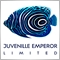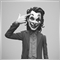# Help with EA Backtest37

Hi,

I am trying to learn how to create a simple robot. Basically as an example I want it to buy when the daily price closes above a MA (144 as example) and then close when the closing prices closes Below the MA, but when I test it I don't get any trades and I get the following

2018.05.24 16:24:56.540 There were 1 passes done during optimization, 1 results have been discarded as insignificant.

Code as below, cant figure out what I am doing wrong, thanks and appreciate the help.

```#include <stdlib.mqh>

#include <stderror.mqh>

//--- indicator settings

#property indicator_chart_window

#property indicator_buffers 2

#property indicator_type1 DRAW_ARROW

#property indicator_width1 1

#property indicator_color1 0xFFAA00

#property indicator_type2 DRAW_ARROW

#property indicator_width2 1

#property indicator_color2 0xFFAA00

//--- indicator buffers

double Buffer1[];

double Buffer2[];

double myPoint; //initialized in OnInit

{

if(type == "print")

Print(message);

else if(type == "error")

{

Print(type+" | Indicator01 @ "+Symbol()+","+Period()+" | "+message);

}

else if(type == "order")

{

}

else if(type == "modify")

{

}

}

//+------------------------------------------------------------------+

//| Custom indicator initialization function                         |

//+------------------------------------------------------------------+

int OnInit()

{

IndicatorBuffers(2);

SetIndexBuffer(0, Buffer1);

SetIndexEmptyValue(0, 0);

SetIndexArrow(0, 241);

SetIndexBuffer(1, Buffer2);

SetIndexEmptyValue(1, 0);

SetIndexArrow(1, 241);

//initialize myPoint

myPoint = Point();

if(Digits() == 5 || Digits() == 3)

{

myPoint *= 10;

}

return(INIT_SUCCEEDED);

}

//+------------------------------------------------------------------+

//| Custom indicator iteration function                              |

//+------------------------------------------------------------------+

int OnCalculate(const int rates_total,

const int prev_calculated,

const datetime& time[],

const double& open[],

const double& high[],

const double& low[],

const double& close[],

const long& tick_volume[],

const long& volume[],

{

int limit = rates_total - prev_calculated;

//--- counting from 0 to rates_total

ArraySetAsSeries(Buffer1, true);

ArraySetAsSeries(Buffer2, true);

//--- initial zero

if(prev_calculated < 1)

{

ArrayInitialize(Buffer1, 0);

ArrayInitialize(Buffer2, 0);

}

else

limit++;

//--- main loop

for(int i = limit-1; i >= 0; i--)

{

if (i >= MathMin(5000-1, rates_total-1-50)) continue; //omit some old rates to prevent "Array out of range" or slow calculation

//Indicator Buffer 1

if(Close[1+i] > iMA(NULL, PERIOD_CURRENT, 144, 0, MODE_SMA, PRICE_CLOSE, i) //Candlestick Close > Moving Average

)

{

Buffer1[i] = Low[i]; //Set indicator value at Candlestick Low

}

else

{

Buffer1[i] = 0;

}

//Indicator Buffer 2

if(Close[1+i] < iMA(NULL, PERIOD_CURRENT, 144, 0, MODE_SMA, PRICE_CLOSE, i) //Candlestick Close < Moving Average

)

{

Buffer2[i] = Low[i]; //Set indicator value at Candlestick Low

}

else

{

Buffer2[i] = 0;

}

}

return(rates_total);

}

```Moderator
10286